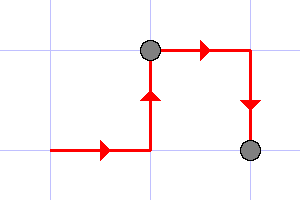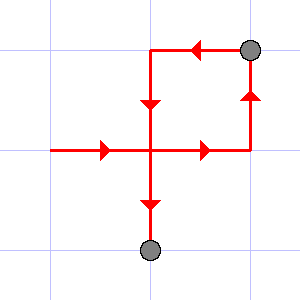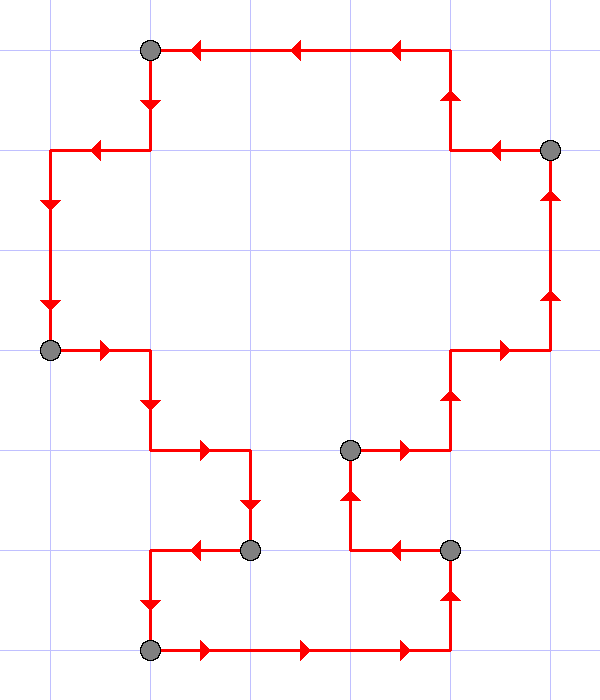# Codeforces Round #389 (Div. 2) C. Santa Claus and Robot 贪心+最短路

C. Santa Claus and Robot
time limit per test
2 seconds
memory limit per test
256 megabytes
input
standard input
output
standard output

Santa Claus has Robot which lives on the infinite grid and can move along its lines. He can also, having a sequence of m pointsp1, p2, ..., pm with integer coordinates, do the following: denote its initial location by p0. First, the robot will move from p0 to p1 along one of the shortest paths between them (please notice that since the robot moves only along the grid lines, there can be several shortest paths). Then, after it reaches p1, it'll move to p2, again, choosing one of the shortest ways, then to p3, and so on, until he has visited all points in the given order. Some of the points in the sequence may coincide, in that case Robot will visit that point several times according to the sequence order.

While Santa was away, someone gave a sequence of points to Robot. This sequence is now lost, but Robot saved the protocol of its unit movements. Please, find the minimum possible length of the sequence.

Input

The first line of input contains the only positive integer n (1 ≤ n ≤ 2·105) which equals the number of unit segments the robot traveled. The second line contains the movements protocol, which consists of n letters, each being equal either L, or R, or U, or Dk-th letter stands for the direction which Robot traveled the k-th unit segment in: L means that it moved to the left, R — to the right, U — to the top and D — to the bottom. Have a look at the illustrations for better explanation.

Output

The only line of input should contain the minimum possible length of the sequence.

Examples
input
```4
RURD
```
output
```2
```
input
```6
RRULDD
```
output
```2
```
input
```26
RRRULURURUULULLLDLDDRDRDLD
```
output
```7
```
input
```3
RLL
```
output
```2
```
input
```4
LRLR
```
output
```4
```
Note

The illustrations to the first three tests are given below.The last example illustrates that each point in the sequence should be counted as many times as it is presented in the sequence.

Source

Codeforces Round #389 (Div. 2, Rated, Based on Technocup 2017 - Elimination Round 3)

My Solution

ans = 0;

(px,py)表示最近出现的1个关键点坐标，cnt表示从最近的那个关键点到当前点所走的路程， (x, y)表示当前所在的点的坐标。

```#include <iostream>
#include <cstdio>
#include <string>
#include <cmath>
using namespace std;
typedef long long LL;
const int maxn = 1e6 + 8;

string s;

int main()
{
#ifdef LOCAL
freopen("c.txt", "r", stdin);
//freopen("c.out", "w", stdout);
int T = 8;
while(T--){
#endif // LOCAL
ios::sync_with_stdio(false); cin.tie(0);

int n, px = 0, py = 0, x = 0, y = 0, rx = 0, ry = 0, ans = 0, cnt = 0;
cin >> n;
cin >> s;
bool flag = false;
for(int i = 0; i < n; i++){
rx = x, ry = y;

if(s[i] == 'L'){
x--;
}
else if(s[i] == 'R'){
x++;
}
else if(s[i] == 'U'){
y++;
}
else if(s[i] == 'D'){
y--;
}

cnt++;
if(abs(px - x) + abs(py - y) == cnt){
//if(!flag){ans++; flag = true; }
}
else{
ans++;
//if(flag) ans++;

cnt = 1;
px = rx;
py = ry;
//cout << px << ' ' << py << endl;

}
}
ans++;
cout << ans << endl;

#ifdef LOCAL
cout << endl;
}
#endif // LOCAL
return 0;
}

```

Thank you!

------from ProLights------分隔线----------------------------

------分隔线----------------------------3粉丝
0关注